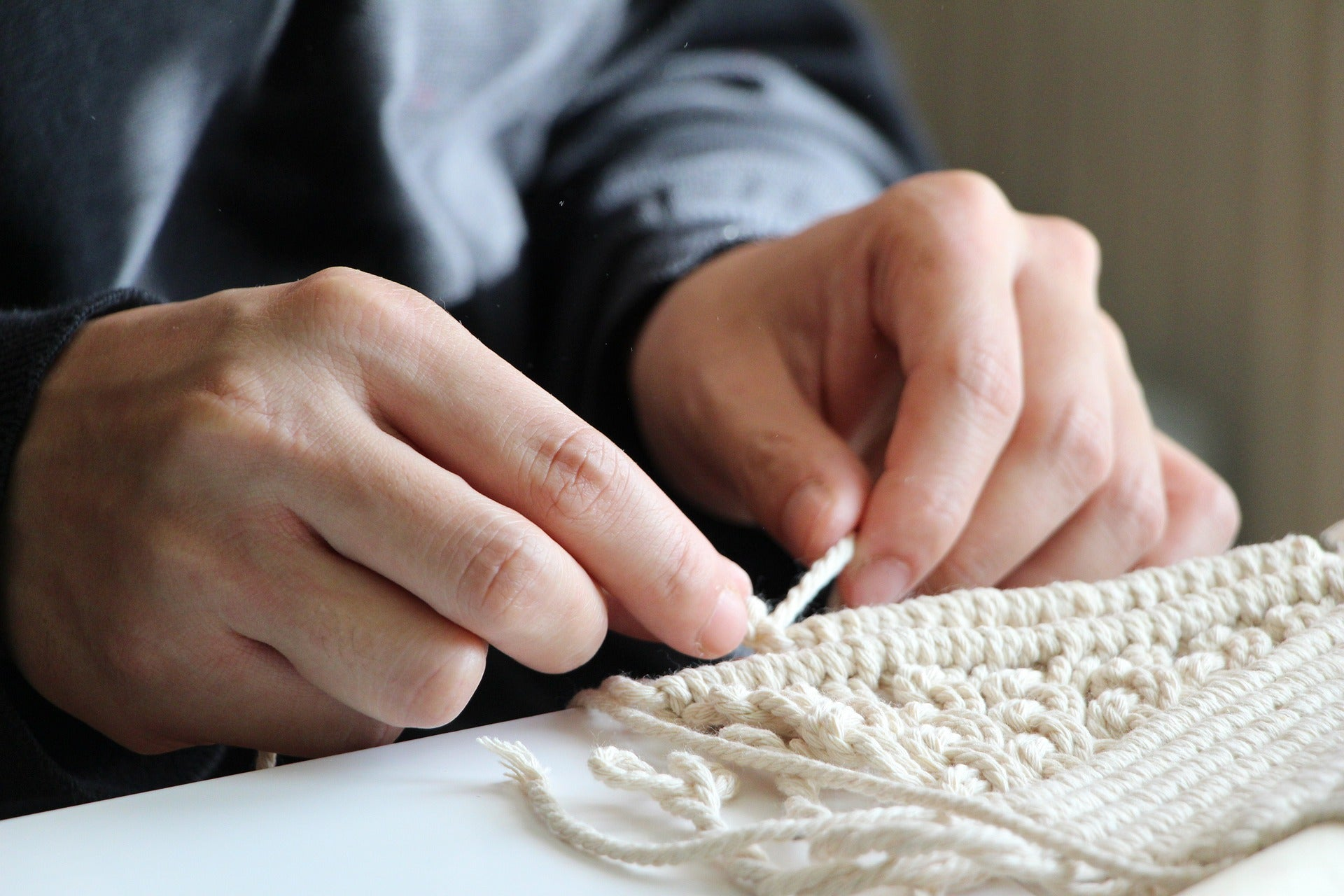# Calculate the length of the threads

## How many macrame ropes do I need?

With a macrame yarn with a diameter of 3mm, which should have a final width of 60cm, i.e. 60 x (0.3 x 3) = 54, you need 54 ropes to make a macrame project.

## How long should the rope be?

Once one knows the size and number of ropes needed, one can calculate the length of threads needed.
If the project contains loose knots or lace patterns, multiply the final length of the project (the longest point) by 4.
If the project consists of tight knots, the length is multiplied by 5. And when using complex knots, the length of the design is multiplied by 6.

Example: For an 80 cm long work that you want to make with 3mm threads, you need 80 x 4 = 320cm or 3.20m for each thread. And thus 3.20 x 54 = 172.8 m thread in total.
Or if the threads are folded in half: 80 x 8 = 640cm or 6.40m for each thread. So 6.40 x 54 = 345.6m thread in total.